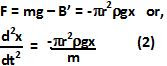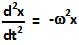## Sunday, July 4, 2010

### Irodov Problem 4.22There are two forces acting on the hydrometer i) the force due to gravity mg pulling it down into the liquid and ii) the buoyant force B acting upwards from the liquid trying to push it out of the liquid. The buoyant force acting on the hydrometer is equal to the weight of the water displaced by the submerged part of the hydrometer.

At equilibrium, the hydrometer is at rest and so B = mg. When the hydrometer is pushed down into the liquid by a distance x beyond the equilibrium point, the hydrometer displaces and additional amount of water and so the Buoyant force increases to B' > B. Thus, the hydrometer is pushed out. Specifically, the additional volume of liquid displaced when the hydrometer is pushed down by a value x isand so the weight of the additional liquid displaced is. Thus,Similarly when the hydrometer is pulled a distance x out of the liquid, the volume of liquid displaced by the hydrometer decreases and so the buoyant force B'' becomes less than B. Thus, the wieght of the hydrometer dominates and pulls the hydrometer back into the liquid.

The net force acting on the hydrometer when its pushed x distance below the equilibrium point is given by,We already know that the equation of motion for a body undergoing simple harmonic motions is given by,whereis the angular frequency. Hence, comparing this with (2) we have,#### 1 comment:

1.